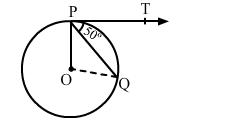# In the given figure, O is the centre of a circle,`
Question:

In the given figure, O is the centre of a circle, PQ is a chord and the tangent PT at P makes an angle of 50° with PQ. Then, ∠POQ = ?(a) 130°
(b) 100°
(c) 90°
(d) 75°

Solution:

(b) $100^{\circ}$

Given, $\angle Q P T=50^{\circ}$

Now, $\angle O P T=90^{\circ}$ (S ince tangents drawn from an external point a re

perpendicular to the radius at point of contact)

$\therefore \angle O P Q=(\angle O P T-\angle Q P T)=\left(90^{\circ}-50^{\circ}\right)=40^{\circ}$

$O P=O Q$ (Radii of the same circle)

$\Rightarrow \angle O P Q=\angle O Q P=40^{0}$

In $\triangle P O Q$,

$\angle P O Q+\angle O P Q+\angle O Q P=180^{\circ}$

$\Rightarrow \angle P O Q+40^{\circ}+40^{0}=180^{0}$

$\Rightarrow \angle P O Q=180^{\circ}-\left(40^{0}+40^{0}\right)$

$\Rightarrow \angle P O Q=180^{\circ}-80^{0}$

$\Rightarrow \angle P O Q=100^{\circ}$